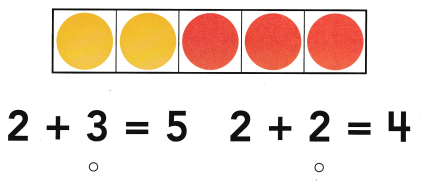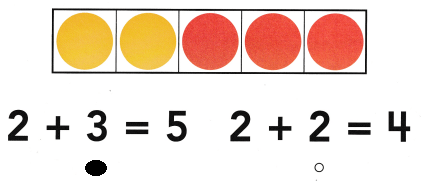Refer to our Texas Go Math Kindergarten Answer Key Pdf to score good marks in the exams. Test yourself by practicing the problems from Texas Go Math Kindergarten Lesson 11.2 Answer Key More Addition.

Explore

DIRECTIONS: Listen to the addition word problem. Circle the set you start with. How many are being added to the set? How many are there now? Trace and write the addition sentence.Explanation:
There are 3 green fishes
and 2 yellow fishes
3 + 2 = 5
the sum of 3 and 2 is 5

Share and Show

DIRECTIONS: 1-3. Listen to the addition word problem. Circle the set you start with. How many are being added to the set? How many are there now? Write and trace the numbers and symbols to complete the addition sentence.

Question 1.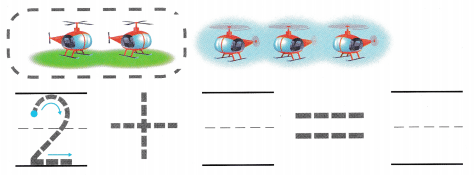Explanation:
There are 2 aero planes in first group
and 3 aero planes in second group
so, there are 5 aero planes in all.
2 + 3 = 5

Question 2.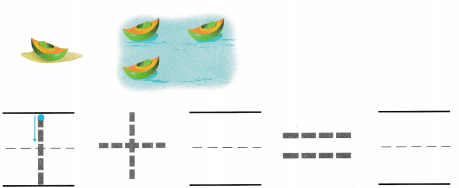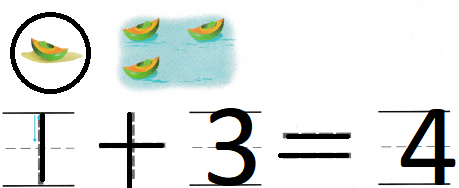Explanation:
In first group there is one ship
and in the second group there is the other ship
so, 1 + 3 = 4
there are 4 ships in all.

Question 3.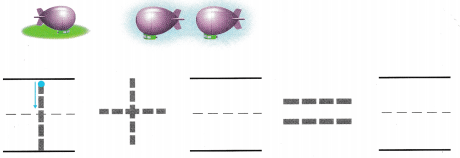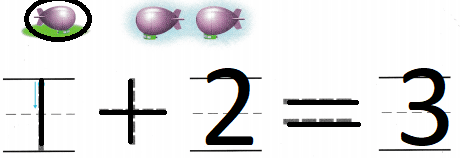Explanation:
In first group there is one toy and
in the second group there is the other toy
so, there are 3 toys in all.

DIRECTIONS: 4-6. Tell an addition word problem about the sets. Circle the set you start with. How many are being added to the set? How many are there now? Write and trace the numbers and symbols to complete the addition sentence.

Question 4.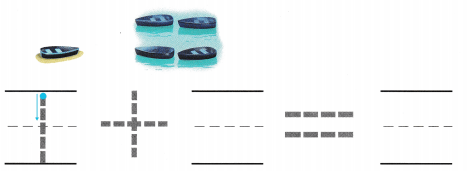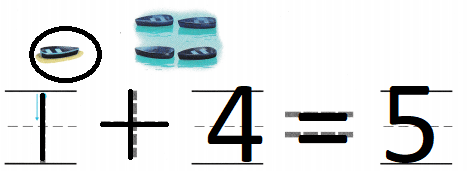Explanation:
In first group there is one boat
and in the second group there are 4 boats
so, 1 + 4 = 5
there are 5 boats in all.

Question 5.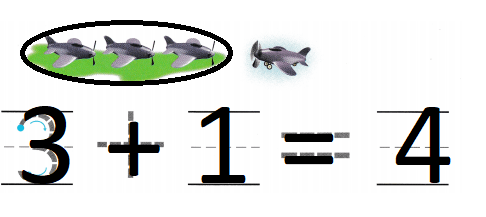Explanation:
There are 3 jets in first group and 1 jet in the second group
so, 3 + 1 = 4
there are 4 jets.

Question 6.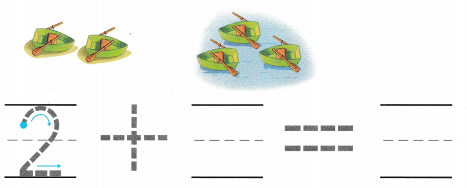Explanation:
In first group there are 2 boats
in the second group there are 3 boats
so, there are 5 boats in all.

HOME ACTIVITY • Have your child show three fingers. Have him or her show more fingers to make five fingers in all. Then have your child tell how many more fingers he or she showed.
3 + 2 = 5
At first he is showing 3 fingers
and to make 5 he has to open 3 more fingers

DIRECTIONS: 7. Bill catches two fish. Jake catches two more fish. How many fish do they catch in all? Draw to show the fish. Trace and write to complete the addition sentence. 8. Choose the correct answer. Which number sentence does the model show?

Problem Solving

Question 7.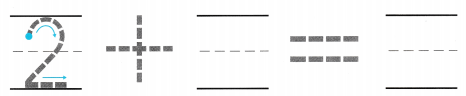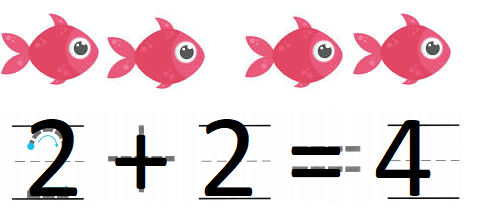Explanation:
Bill catches two fish.
Jake catches two more fish.
2 + 2 = 4
so, there are 4 fishes in all.

Question 8.Explanation:
In a five frame there are 3 red counters
and 2 yellow counters
so, there are 54 counters in all.

### Texas Go Math Kindergarten Lesson 11.2 Homework and Practice Answer Key

DIRECTIONS: Listen to the addition word problem. Circle the set you start with. Trace and write to complete the addition sentence. 1. Two kittens are playing. Two more kittens come. How many kittens are there now? 2. Two chicks are eating. One more chick comes. How many chicks are there now?

Question 1.Explanation:
Two kittens are playing.
Two more kittens come.
2 + 2 = 4
so, there are 4 kittens.

Question 2.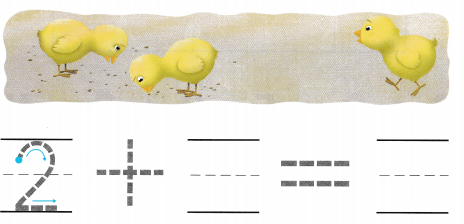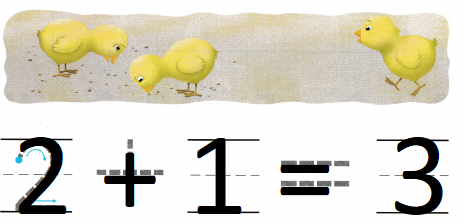Explanation:
Two chicks are eating.
One more chick comes.
2 + 1 = 3
so, there are 3 chicks

DIRECTIONS: Choose the correct answer. 3-4. Which number sentence does the model show?

Lesson Check

Question 3.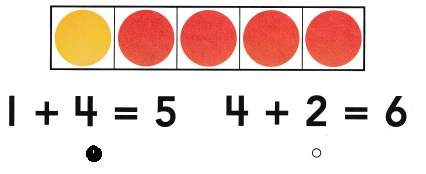Explanation:
In a five frame there are 4 red counters and
1 yellow counter
so, there are 5 counters in all.

Question 4.